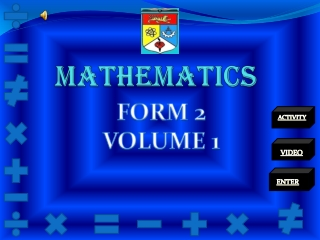DownloadDownload PresentationMATHEMATICS

# MATHEMATICS

Télécharger la présentation## MATHEMATICS

- - - - - - - - - - - - - - - - - - - - - - - - - - - E N D - - - - - - - - - - - - - - - - - - - - - - - - - - -
##### Presentation Transcript

1. MATHEMATICS FORM 2 VOLUME 1 ACTIVITY VIDEO ENTER

2. CHAPTER 1 DIRECTED NUMBERS 1.1 Multiplication and division of integers 1.2 Combined operations ( Addition, Subtraction, Multiplication and Division ) 1.3 Positive and Negative Fractions 1.4 Positive and Negative Decimals 1.5 Computations involving Directed Numbers NEXT

3. KEYWORDS REMEMBER THIS !!! • POSITIVE SIGN (+) • NEGATIVE SIGN (-) • ADDITION (+) • SUBTRACTION (-) • GREATER THAN (>) • LESS THAN (<) NEXT

4. 1.1 MULTIPLICATION AND DIVISION OF INTEGERS Learning Outcome : a) Multiply integers and solve problems involving of multiplication of integers. b) Divide integers and solve problems involving division of integers. The positive numbers such as 1, 2, 3, 4, …. are positive integers. The negative numbers such as -1, -2, -3, -4, …. are negative integers THE PRODUCT /QUOTIENT OF TWO INTEGERS WITH LIKE SIGNS IS +VE INTEGERS THE PRODUCT/QUOTIENT OF TWO INTEGERS WITH UNLIKE SIGNS IS –VE INTEGERS NEXT

5. EXAMPLE FOR THIS SUB TOPIC 1) 6×(-7) × (-2)TIPS !!! Solution: the +ve sign is usually =6×(14) omitted =84 +30 is written as 30 2)-27 ÷ 9 ÷ 3 !!! Solution: a×b=b×a =-3 ÷ 3 =-1 NEXT

6. 1.2 COMBINATION OPERATION ( + - × ÷ ) REMEMBER!!! B O D M A S ( ) of ÷ × + - To perform computations involving combined operations, you must abide by BODMAS rule. Calculation within brackets must be performed first, followed by division or multiplication, then addition or subtraction, from left to right. Eg: 125 ÷ (-5) + 6 × (-2) SOLUTION: = ( -25 ) + ( - 12 ) • Solve the division or multiplication = - 37 first 2) Then solve the addition NEXT

7. 1.3 POSITIVE AND NEGATIVE FRACTION • +VE (POSITIVE FRACTION) A fraction with a value greater than zero ( > 0) is a positive fraction eg: ½, 2/5, 6/5, 1/6, … • -VE (NEGATIVE FRACTION) A fraction with a value less than zero ( < 0 ) is a negative fraction eg: -1/3, -5/6, -9/10, -4/7, … COMPARE AND ORDER FRACTION <0 >0 --------l---------l---------l---------l---------l----------l---------l------- -3/4 -1/2 -1/4 0 ¼ ½ ¾ -ve fraction zero +ve fraction NEXT

8. A fraction is always greater than the fraction to its left For example : ½ is greater than ¼ -1/4 is greater than -1/2 ¼ is greater than -1/4 • A fraction is always less than the fraction to its right For example : ¼ is less than ½ -1/2 is less than -1/4 -1/4 is less than ¼ TIPS + (+a) = +a -(-a) = +a SEE THE + (-a) = -a -(+a) = -a SYMBOL CAREFULLY NEXT

9. EXAMPLE FOR THIS SUB TOPIC • Arrange the following fractions in ascending and descending order 1/6, -1/3, -3/8, 1/8, -3/4 Solution : 1/6, -1/3, -3/8, 1/8, -3/4 The LCM of 3, 4, 6, and 8 is 24 Change the fraction to their respective equivalent fractions with the denominator of 24 Ascending order : -3/4, -3/8, -1/3, 1/8, 1/6 Descending order : 1/6, 1/8, -1/3, -3/8, -3/4 NEXT

10. 1.4 POSITIVE AND NEGATIVE DECIMALS • A decimal with a value greater than zero is apositive decimal such as 0.21, 7.89, 9.54, 8.88, … • A decimal with a value less than zero is a negativedecimalsuch as -0.05, -4.45, -9.90, -3.54, … COMPARE AND ORDER DECIMAL ---l------------l-------------l-------------l------------l--- -2.22 -1.11 o.00 1.11 2.22 negative decimal zero positive decimal DID YOUascending means increasing KNOW???Descending means decreasing NEXT

11. 1.5 COMPUTATIONS INVOLVING DIRECTED NUMBERS • As integers, computation involving directed numbers follow the BODMAS rule. • Involving two directed numbers Eg: 4/5 × (8.2) solution: = - (4/5 × 82/10) = -328/50 = - 6/14/25 • Involving the combination of two or more operations on directed numbers Eg: [ 55 + (-37)] × 6 solution: = 18 × 6 = 108 BACK If you want see video please click “BACK”

12. LET SEE THIS VIDEO… CONTINUE More video

13. SUMMARY NEXT

14. SKILL CHECKLIST • Can you multiply and divide integers? • Can you solve problem involving multiplication and division of integers? • Can you perform computation and solve the problem involving combined operation of integers? • Can you compare, order and perform computation involving fraction and decimals? • Can you perform computations involving combined operations of directed numbers? • Can you pose and solve problems involving directed numbers. CLICK “BACK” TO DO ACTIVITY BACK

15. EXERCISE CLICK “exercise” BELOW TO DO MORE EXERCISES EXERCISE END

16. THE END OF THIS TOPIC THANK YOU PREPARED BY: NORMIRA BINTI HJ IBRAHIM (A 132219) Student of Mathematics’ Education National University of Malaysia THANK YOU FOR YOUR ATTENTION